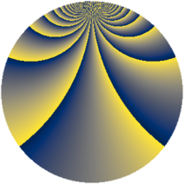# Properties

 Label 2760.2.dmLevel $2760$ Weight $2$ Character orbit 2760.dm Rep. character $\chi_{2760}(97,\cdot)$ Character field $\Q(\zeta_{44})$ Dimension $1440$ Sturm bound $1152$

# Related objects

## Defining parameters

 Level: $$N$$ $$=$$ $$2760 = 2^{3} \cdot 3 \cdot 5 \cdot 23$$ Weight: $$k$$ $$=$$ $$2$$ Character orbit: $$[\chi]$$ $$=$$ 2760.dm (of order $$44$$ and degree $$20$$) Character conductor: $$\operatorname{cond}(\chi)$$ $$=$$ $$115$$ Character field: $$\Q(\zeta_{44})$$ Sturm bound: $$1152$$

## Dimensions

The following table gives the dimensions of various subspaces of $$M_{2}(2760, [\chi])$$.

Total New Old
Modular forms 11840 1440 10400
Cusp forms 11200 1440 9760
Eisenstein series 640 0 640

## Trace form

 $$1440q + O(q^{10})$$ $$1440q + 24q^{23} - 16q^{25} + 16q^{31} - 88q^{37} + 48q^{41} - 160q^{47} + 32q^{55} - 88q^{57} - 16q^{73} + 32q^{75} + 96q^{77} + 144q^{81} + 200q^{85} + 48q^{87} - 48q^{93} + 416q^{95} + O(q^{100})$$

## Decomposition of $$S_{2}^{\mathrm{new}}(2760, [\chi])$$ into newform subspaces

The newforms in this space have not yet been added to the LMFDB.

## Decomposition of $$S_{2}^{\mathrm{old}}(2760, [\chi])$$ into lower level spaces

$$S_{2}^{\mathrm{old}}(2760, [\chi]) \cong$$ $$S_{2}^{\mathrm{new}}(115, [\chi])$$$$^{\oplus 8}$$$$\oplus$$$$S_{2}^{\mathrm{new}}(230, [\chi])$$$$^{\oplus 6}$$$$\oplus$$$$S_{2}^{\mathrm{new}}(345, [\chi])$$$$^{\oplus 4}$$$$\oplus$$$$S_{2}^{\mathrm{new}}(460, [\chi])$$$$^{\oplus 4}$$$$\oplus$$$$S_{2}^{\mathrm{new}}(690, [\chi])$$$$^{\oplus 3}$$$$\oplus$$$$S_{2}^{\mathrm{new}}(920, [\chi])$$$$^{\oplus 2}$$$$\oplus$$$$S_{2}^{\mathrm{new}}(1380, [\chi])$$$$^{\oplus 2}$$